Adding and Subtracting Rational Expressions:
Examples
(page 2 of 3)As you follow along in these examples, note how I do everything neatly and orderly. You should model your homework after these exercises, to help minimize errors.

• Simplify the following:
•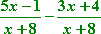These fractions already have a common denominator, so I can just add. But I'll use parentheses on the numerators, to make sure I carry the "minus" through the second parentheses. (A common mistake would be to take the "minus" sign only onto the "3x" and not onto the "4".)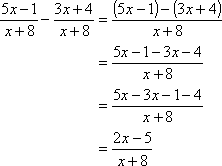Then the answer is: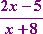• Simplify the following:
•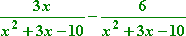Again, these already have a common denominator, so I can just combine them as they are. But the denominator is a quadratic, so I'll want to factor the numerator when I'm done, to check and see if anything cancels out.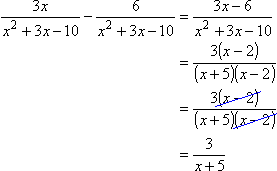As you can see, something did cancel. You always need to remember this step: factor the denominator and numerator (if possible) and check for common factors. By the way, since I was able to cancel off the "x – 2" factor, this eliminated a zero from the denominator. Depending on your book and on your instructor, you may (or may not) need to account for this change in the domain of the fraction. Copyright © Elizabeth Stapel 2003-2011 All Rights Reserved

Advertisement

The complete answer is: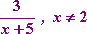Depending on your instructor, you might not need the "for x not equal to 2" part. If you're not sure, ask now, before the test.

• Simplify the following:
•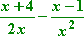First I have to convert these fractions to the common denominator of 2x2. (If you're not sure about the common denominator, do the factor table, as shown in the second example on the previous page, to check.) Then I'll add and, if possible, cancel off common factors.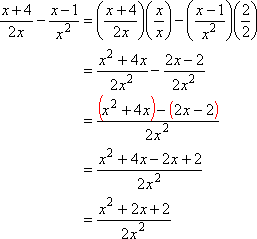Note how I used parentheses to keep my subtraction straight. I wanted to be sure to carry the "minus" through properly, and the extra step with the parentheses is very helpful for this. Nothing cancelled in this case, so the answer is: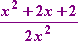It isn't common that you will be able to simplify a rational addition or subtraction problem, but you should get in the habit of checking. I would bet good money that you'll have a problem that simplifies on the test.

• Simplify the following:
•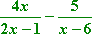The two denominators have no common factors, so the common denominator will be
(2x – 1)(x – 6).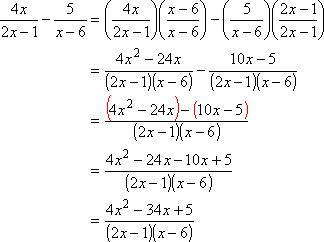The numerator doesn't factor, so there is no chance of anything cancelling off. It is customary to leave the denominator factored like this, so, unless your instructor says otherwise, don't bother multiplying the denominator out. The answer is: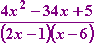• Simplify the following:
•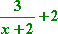Don't let this one throw you. The denominator of the "2" is just "1", so the common denominator will be the only other denominator of interest: "x + 2".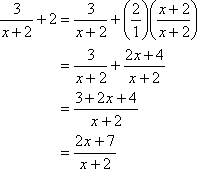Nothing cancels, so the answer is: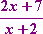<< Previous  Top  |  1 | 2 | 3  |  Return to Index  Next >>

 Cite this article as: Stapel, Elizabeth. "Adding and Subtracting Rational Expressions: Examples." Purplemath. Available from https://www.purplemath.com/modules/rtnladd2.htm. Accessed [Date] [Month] 2016

MathHelp.com Courses
 Copyright © 2021  Elizabeth Stapel   |   About   |   Terms of Use   |   Linking   |   Site Licensing Contact Us